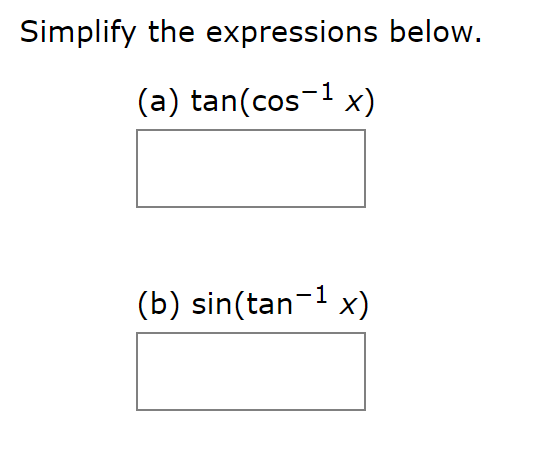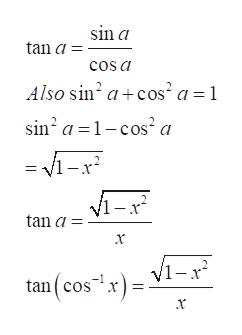Simplify the expressions below.(a) tan(cos-1 x)(b) sin(tan1 x)

Questionhelp_outlineImage TranscriptioncloseSimplify the expressions below. (a) tan(cos-1 x) (b) sin(tan1 x) fullscreen
Step 1

Refer to the question.

Let a = cos^(-1) x.  That is from the properties of inverse trigonometric,

Step 2

Now tan(cos^(-1) x)= tan(a)help_outlineImage Transcriptionclosesin a tan a cos a Also sin2 a+cos2 a 1 sin a1-cos2 a tan a tan(cos= V1-r fullscreen
Step 3

Now for part b sin(...

Want to see the full answer?

See Solution

Want to see this answer and more?

Our solutions are written by experts, many with advanced degrees, and available 24/7

See Solution
Tagged in

Calculus